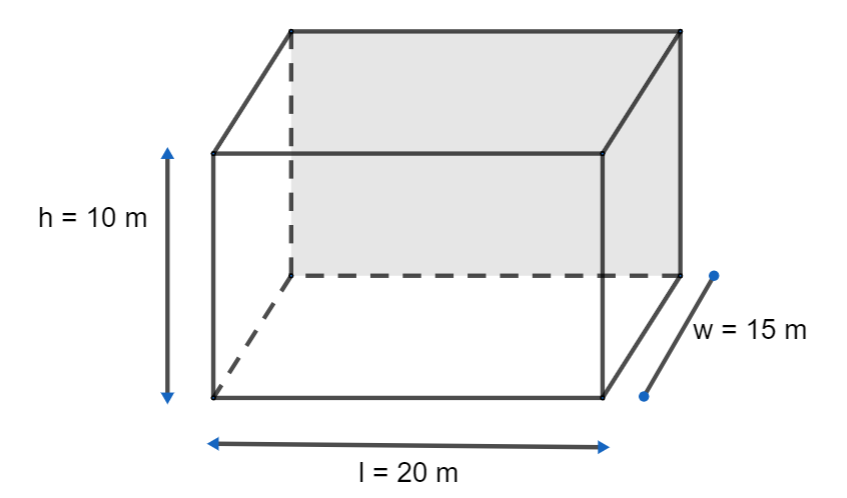Courses
Courses for Kids
Free study material
Offline Centres
MoreLast updated date: 04th Dec 2023
Total views: 281.7k
Views today: 5.81k

# A restaurant hall is 20 metres long, 15 metres wide and 10 metres high. The interior of the hall except the ceiling and the base has to be covered with a bright wallpaper. What will be the total expenditure if the wall costs Rs.60 per square metre?Verified
281.7k+ views
Hint: In this problem, we have to find the total expenditure of the wallpaper if the wall costs Rs.60 per square metre, where we are given the length 20 metres, 15 metres wide and 10 metres height. We are also given that the bright wallpaper can be used except for the ceiling and the base, so we can subtract the area of the ceiling and the area of the base from the total surface area of all face and multiply the cost per square metres to get the final answer.

Complete step-by-step solution:
We are given a restaurant hall of length 20 metres, 15 metres wide and 10 metres height.We are also given that the bright wallpaper can be used except for the ceiling and the base.
So, we can subtract the area of the ceiling and the area of the base from the total surface area of all faces.
We can now find the total surface area of all faces, with the formula area of the cuboid,$2\left( lb+bh+hl \right)$.
We can now substitute the values in the above formula, we get
$\Rightarrow 2\left( 20\times 15+15\times 10+10\times 20 \right)$
We can now simplify the above step, we get
$\Rightarrow 2\left( 300+150+200 \right)=1300$square metres.
We can now find the area of the ceiling (area of rectangle) with the formula $l\times b$.
$\Rightarrow 20\times 15=300$square metres.
Where the area of the ceiling = area of the base = 300 square metres.
We can now subtract the total surface area of all side and the area of ceiling + are of base, we get
$\Rightarrow 1300-\left( 300+300 \right)=700$square metres.
The interior area of the restaurant except the ceiling and the base = 700 square metres.
We know that the cost of wallpaper per square metre is Rs.60.
We can now multiply it with the interior area of the restaurant except the ceiling and the base, we get
$\Rightarrow 700\times 60=42000$
Therefore, the total expenditure is Rs.42000.

Note: We should always remember that the formula for the total surface area of a cuboid is $2\left( lb+bh+hl \right)$, where the restaurant hall will be in a cuboid form. We should also remember the area of one side of the cuboid that is a rectangle is $l\times b$, where l is the length, b is the breadth and h is the height.